Function Repository Resource:

# ChordalGraphQ

Test whether a graph is chordal

Contributed by: Jon McLoone
 ResourceFunction["ChordalGraphQ"][gr] test whether the graph gr is chordal.

## Details

A chordal graph is one in which all cycles of four or more vertices have a chord, which is an edge that is not part of the cycle but connects two vertices of the cycle.

## Examples

### Basic Examples (3)

Complete graphs are chordal:

 In:=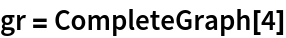Out=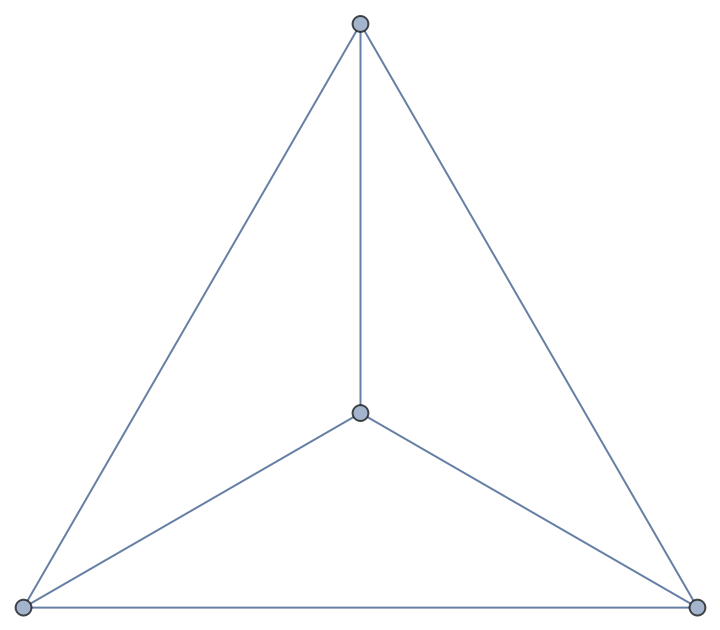In:=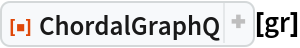Out=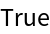The HouseX graph is chordal:

 In:=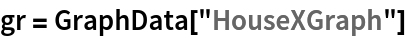Out=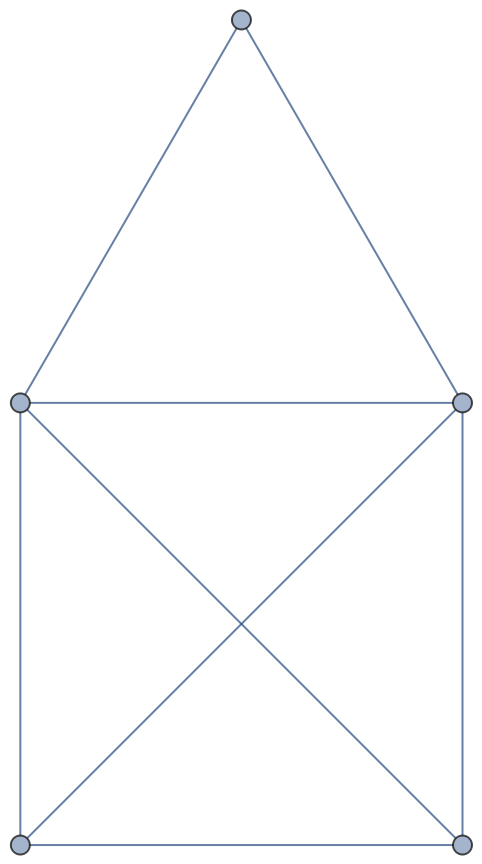In:=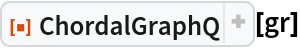Out=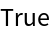But the house graph is not:

 In:=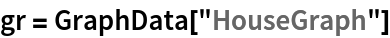Out=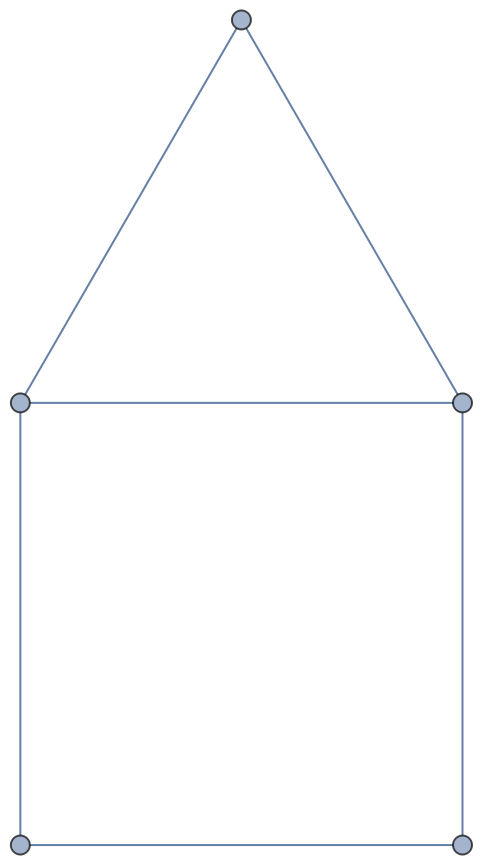In:=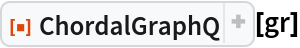Out=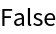This simple cycle is not chordal:

 In:=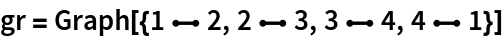Out=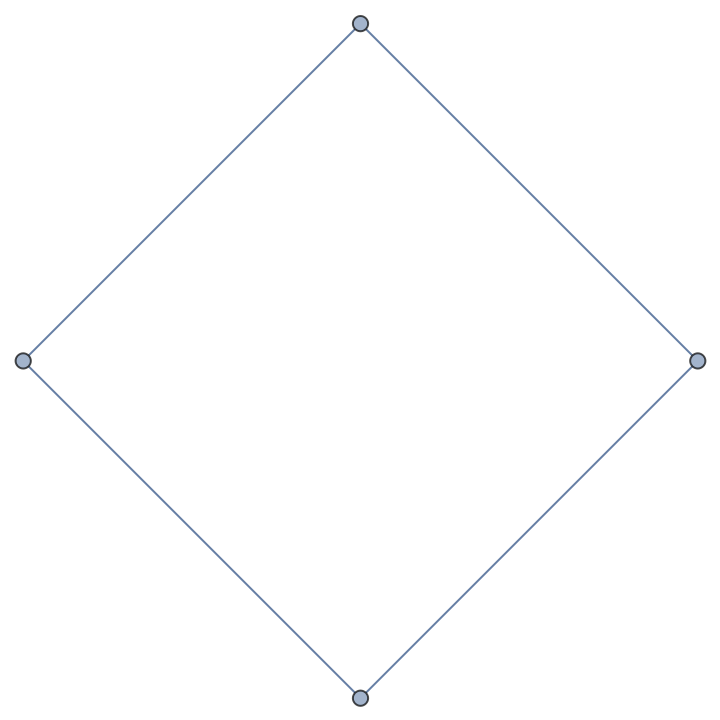In:=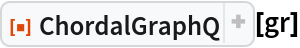Out=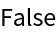Jon McLoone

## Version History

• 1.0.0 – 25 March 2022